## Logistic Growth, S-Curves, Bifurcations, & Lyapunov Exponents in R (Tidyversion)

This is an updated version of my 2015 (Base R) blog post using tidyverse. Original Rmd is available on request.

## Imperfect Action is Better Than Perfect Inaction: What Harry Truman Can Teach Us About Loss Functions (with an intro to ggplot)One of the heuristics we use at Intelex to guide decision making is former US President Truman’s advice that “imperfect action is better than perfect inaction.” What it means is — don’t wait too long to take action, because you don’t want to miss opportunities. Good advice, right?

When I share this with colleagues, I often hear a response like: “that’s dangerous!” To which my answer is “well sure, sometimes, but it can be really valuable depending on how you apply it!” The trick is: knowing how and when.

Here’s how it can be dangerous. For example, statistical process control (SPC) exists to keep us from tampering with processes — from taking imperfect action based on random variation, which will not only get us nowhere, but can exacerbate the problem we were trying to solve. The secret is to apply Truman’s heuristic based on an understanding of exactly how imperfect is OK with your organization, based on your risk appetite. And this is where loss functions can help.

Along the way, we’ll demonstrate how to do a few important things related to plotting with the ggplot package in R, gradually adding in new elements to the plot so you can see how it’s layered, including:

• Plot a function based on its equation
• Add text annotations to specific locations on a ggplot
• Draw horizontal and vertical lines on a ggplot
• Draw arrows on a ggplot
• Add extra dots to a ggplot
• Eliminate axis text and axis tick marks

## What is a Loss Function?

A loss function quantifies how unhappy you’ll be based on the accuracy or effectiveness of a prediction or decision. In the simplest case, you control one variable (x) which leads to some cost or loss (y). For the case we’ll examine in this post, the variables are:

• How much time and effort you put in to scoping and characterizing the problem (x); we assume that time+effort invested leads to real understanding
• How much it will cost you (y); can be expressed in terms of direct costs (e.g. capex + opex) as well as opportunity costs or intangible costs (e.g. damage to reputation)

Here is an example of what this might look like, if you have a situation where overestimating (putting in too much x) OR underestimating (putting in too little x) are both equally bad. In this case, x=10 is the best (least costly) decision or prediction:

``````# describe the equation we want to plot
parabola <- function(x) ((x-10)^2)+10

# initialize ggplot with a dummy dataset
library(ggplot)
p <- ggplot(data = data.frame(x=0), mapping = aes(x=x))

p + stat_function(fun=parabola) + xlim(-2,23) + ylim(-2,100) +
xlab("x = the variable you can control") +
ylab("y = cost of loss (\$\$)")``````

In regression (and other techniques where you’re trying to build a model to predict a quantitative dependent variable), mean square error is a squared loss function that helps you quantify error. It captures two facts: the farther away you are from the correct answer the worse the error is — and both overestimating and underestimating is bad (which is why you square the values). Across this and related techniques, the loss function captures these characteristics:

Not all loss functions have that general shape. For classification, for example, the 0-1 loss function tells the story that if you get a classification wrong (x < 0) you incur all the penalty or loss (y=1), whereas if you get it right (x > 0) there is no penalty or loss (y=0):

``````# set up data frame of red points
d.step <- data.frame(x=c(-3,0,0,3), y=c(1,1,0,0))

# note that the loss function really extends to x=-Inf and x=+Inf
ggplot(d.step) + geom_step(mapping=aes(x=x, y=y), direction="hv") +
geom_point(mapping=aes(x=x, y=y), color="red") +
xlab("y* f(x)") + ylab("Loss (Cost)") +
ggtitle("0-1 Loss Function for Classification")``````

## Use the Loss Function to Make Strategic Decisions

So let’s get back to Truman’s advice. Ideally, we want to choose the x (the amount of time and effort to invest into project planning) that results in the lowest possible cost or loss. That’s the green point at the nadir of the parabola:

``````p + stat_function(fun=parabola) + xlim(-2,23) + ylim(-2,100) +
xlab("Time Spent and Information Gained (e.g. person-weeks)") + ylab("\$\$ COST \$\$") +
annotate(geom="text", x=10, y=5, label="Some Effort, Lowest Cost!!", color="darkgreen") +
geom_point(aes(x=10, y=10), colour="darkgreen")``````

Costs get higher as we move up the x-axis:

``````p + stat_function(fun=parabola) + xlim(-2,23) + ylim(-2,100) +
xlab("Time Spent and Information Gained (e.g. person-weeks)") + ylab("\$\$ COST \$\$") +
annotate(geom="text", x=10, y=5, label="Some Effort, Lowest Cost!!", color="darkgreen") +
geom_point(aes(x=10, y=10), colour="darkgreen") +
annotate(geom="text", x=0, y=100, label="\$\$\$\$\$", color="green") +
annotate(geom="text", x=0, y=75, label="\$\$\$\$", color="green") +
annotate(geom="text", x=0, y=50, label="\$\$\$", color="green") +
annotate(geom="text", x=0, y=25, label="\$\$", color="green") +
annotate(geom="text", x=0, y=0, label="\$ 0", color="green")``````

And time+effort grows as we move along the x-axis (we might spend minutes on a problem at the left of the plot, or weeks to years by the time we get to the right hand side):

``````p + stat_function(fun=parabola) + xlim(-2,23) + ylim(-2,100) +
xlab("Time Spent and Information Gained (e.g. person-weeks)") + ylab("\$\$ COST \$\$") +
annotate(geom="text", x=10, y=5, label="Some Effort, Lowest Cost!!", color="darkgreen") +
geom_point(aes(x=10, y=10), colour="darkgreen") +
annotate(geom="text", x=0, y=100, label="\$\$\$\$\$", color="green") +
annotate(geom="text", x=0, y=75, label="\$\$\$\$", color="green") +
annotate(geom="text", x=0, y=50, label="\$\$\$", color="green") +
annotate(geom="text", x=0, y=25, label="\$\$", color="green") +
annotate(geom="text", x=0, y=0, label="\$ 0", color="green") +
annotate(geom="text", x=2, y=0, label="minutes\nof effort", size=3) +
annotate(geom="text", x=20, y=0, label="months\nof effort", size=3)``````

## Planning too Little = Planning too Much = Costly

What this means is — if we don’t plan, or we plan just a little bit, we incur high costs. We might make the wrong decision! Or miss critical opportunities! But if we plan too much — we’re going to spend too much time, money, and/or effort compared to the benefit of the solution we provide.

``````p + stat_function(fun=parabola) + xlim(-2,23) + ylim(-2,100) +
xlab("Time Spent and Information Gained (e.g. person-weeks)") + ylab("\$\$ COST \$\$") +
annotate(geom="text", x=10, y=5, label="Some Effort, Lowest Cost!!", color="darkgreen") +
geom_point(aes(x=10, y=10), colour="darkgreen") +
annotate(geom="text", x=0, y=100, label="\$\$\$\$\$", color="green") +
annotate(geom="text", x=0, y=75, label="\$\$\$\$", color="green") +
annotate(geom="text", x=0, y=50, label="\$\$\$", color="green") +
annotate(geom="text", x=0, y=25, label="\$\$", color="green") +
annotate(geom="text", x=0, y=0, label="\$ 0", color="green") +
annotate(geom="text", x=2, y=0, label="minutes\nof effort", size=3) +
annotate(geom="text", x=20, y=0, label="months\nof effort", size=3) +
annotate(geom="text",x=3, y=85, label="Little (or no) Planning\nHIGH COST", color="red") +
annotate(geom="text", x=18, y=85, label="Paralysis by Planning\nHIGH COST", color="red") +
geom_vline(xintercept=0, linetype="dotted") + geom_hline(yintercept=0, linetype="dotted")``````

The trick is to FIND THAT CRITICAL LEVEL OF TIME and EFFORT invested to gain information and understanding about your problem… and then if you’re going to err, make sure you err towards the left — if you’re going to make a mistake, make the mistake that costs less and takes less time to make:

``````arrow.x <- c(10, 10, 10, 10)
arrow.y <- c(35, 50, 65, 80)
arrow.x.end <- c(6, 6, 6, 6)
arrow.y.end <- arrow.y
d <- data.frame(arrow.x, arrow.y, arrow.x.end, arrow.y.end)

p + stat_function(fun=parabola) + xlim(-2,23) + ylim(-2,100) +
xlab("Time Spent and Information Gained (e.g. person-weeks)") + ylab("\$\$ COST \$\$") +
annotate(geom="text", x=10, y=5, label="Some Effort, Lowest Cost!!", color="darkgreen") +
geom_point(aes(x=10, y=10), colour="darkgreen") +
annotate(geom="text", x=0, y=100, label="\$\$\$\$\$", color="green") +
annotate(geom="text", x=0, y=75, label="\$\$\$\$", color="green") +
annotate(geom="text", x=0, y=50, label="\$\$\$", color="green") +
annotate(geom="text", x=0, y=25, label="\$\$", color="green") +
annotate(geom="text", x=0, y=0, label="\$ 0", color="green") +
annotate(geom="text", x=2, y=0, label="minutes\nof effort", size=3) +
annotate(geom="text", x=20, y=0, label="months\nof effort", size=3) +
annotate(geom="text",x=3, y=85, label="Little (or no) Planning\nHIGH COST", color="red") +
annotate(geom="text", x=18, y=85, label="Paralysis by Planning\nHIGH COST", color="red") +
geom_vline(xintercept=0, linetype="dotted") +
geom_hline(yintercept=0, linetype="dotted") +
geom_vline(xintercept=10) +
geom_segment(data=d, mapping=aes(x=arrow.x, y=arrow.y, xend=arrow.x.end, yend=arrow.y.end),
arrow=arrow(), color="blue", size=2) +
annotate(geom="text", x=8, y=95, size=2.3, color="blue",
label="we prefer to be\non this side of the\nloss function")``````

## Moral of the Story

The moral of the story is… imperfect action can be expensive, but perfect action is ALWAYS expensive. Spend less to make mistakes and learn from them, if you can! This is one of the value drivers for agile methodologies… agile practices can help improve communication and coordination so that the loss function is minimized.

``````## FULL CODE FOR THE COMPLETELY ANNOTATED CHART ##
# If you change the equation for the parabola, annotations may shift and be in the wrong place.
parabola <- function(x) ((x-10)^2)+10

my.title <- expression(paste("Imperfect Action Can Be Expensive. But Perfect Action is ", italic("Always"), " Expensive."))

arrow.x <- c(10, 10, 10, 10)
arrow.y <- c(35, 50, 65, 80)
arrow.x.end <- c(6, 6, 6, 6)
arrow.y.end <- arrow.y
d <- data.frame(arrow.x, arrow.y, arrow.x.end, arrow.y.end)

p + stat_function(fun=parabola) + xlim(-2,23) + ylim(-2,100) +
xlab("Time Spent and Information Gained (e.g. person-weeks)") + ylab("\$\$ COST \$\$") +
annotate(geom="text", x=10, y=5, label="Some Effort, Lowest Cost!!", color="darkgreen") +
geom_point(aes(x=10, y=10), colour="darkgreen") +
annotate(geom="text", x=0, y=100, label="\$\$\$\$\$", color="green") +
annotate(geom="text", x=0, y=75, label="\$\$\$\$", color="green") +
annotate(geom="text", x=0, y=50, label="\$\$\$", color="green") +
annotate(geom="text", x=0, y=25, label="\$\$", color="green") +
annotate(geom="text", x=0, y=0, label="\$ 0", color="green") +
annotate(geom="text", x=2, y=0, label="minutes\nof effort", size=3) +
annotate(geom="text", x=20, y=0, label="months\nof effort", size=3) +
annotate(geom="text",x=3, y=85, label="Little (or no) Planning\nHIGH COST", color="red") +
annotate(geom="text", x=18, y=85, label="Paralysis by Planning\nHIGH COST", color="red") +
geom_vline(xintercept=0, linetype="dotted") +
geom_hline(yintercept=0, linetype="dotted") +
geom_vline(xintercept=10) +
geom_segment(data=d, mapping=aes(x=arrow.x, y=arrow.y, xend=arrow.x.end, yend=arrow.y.end),
arrow=arrow(), color="blue", size=2) +
annotate(geom="text", x=8, y=95, size=2.3, color="blue",
label="we prefer to be\non this side of the\nloss function") +
ggtitle(my.title) +
theme(axis.text.x=element_blank(), axis.ticks.x=element_blank(),
axis.text.y=element_blank(), axis.ticks.y=element_blank()) ``````

Now sometimes you need to make this investment! (Think nuclear power plants, or constructing aircraft carriers or submarines.) Don’t get caught up in getting your planning investment perfectly optimized — but do be aware of the trade-offs, and go into the decision deliberately, based on the risk level (and regulatory nature) of your industry, and your company’s risk appetite.

## A Discrete Time Markov Chain (DTMC) SIR Model in RImage Credit: Doug Buckley of http://hyperactive.to

There are many different techniques that be used to model physical, social, economic, and conceptual systems. The purpose of this post is to show how the Kermack-McKendrick (1927) formulation of the SIR Model for studying disease epidemics (where S stands for Susceptible, I stands for Infected, and R for Recovered) can be easily implemented in R as a discrete time Markov Chain using the markovchain package.

A Discrete Time Markov Chain (DTMC) is a model for a random process where one or more entities can change state between distinct timesteps. For example, in SIR, people can be labeled as Susceptible (haven’t gotten a disease yet, but aren’t immune), Infected (they’ve got the disease right now), or Recovered (they’ve had the disease, but no longer have it, and can’t get it because they have become immune). If they get the disease, they change states from Susceptible to Infected. If they get well, they change states from Infected to Recovered. It’s impossible to change states between Susceptible and Recovered, without first going through the Infected state. It’s totally possible to stay in the Susceptible state between successive checks on the population, because there’s not a 100% chance you’ll actually be infected between any two timesteps. You might have a particularly good immune system, or maybe you’ve been hanging out by yourself for several days programming.

Discrete time means you’re not continuously monitoring the state of the people in the system. It would get really overwhelming if you had to ask them every minute “Are you sick yet? Did you get better yet?” It makes more sense to monitor individuals’ states on a discrete basis rather than continuously, for example, like maybe once a day. (Ozgun & Barlas (2009) provide a more extensive illustration of the difference between discrete and continuous modeling, using a simple queuing system.)

To create a Markov Chain in R, all you need to know are the 1) transition probabilities, or the chance that an entity will move from one state to another between successive timesteps, 2) the initial state (that is, how many entities are in each of the states at time t=0), and 3) the markovchain package in R. Be sure to install markovchain before moving forward.

Imagine that there’s a 10% infection rate, and a 20% recovery rate. That implies that 90% of Susceptible people will remain in the Susceptible state, and 80% of those who are Infected will move to the Recovered Category, between successive timesteps. 100% of those Recovered will stay recovered. None of the people who are Recovered will become Susceptible.

Say that you start with a population of 100 people, and only 1 is infected. That means your “initial state” is that 99 are Susceptible, 1 is Infected, and 0 are Recovered. Here’s how you set up your Markov Chain:

```library(markovchain)
mcSIR <- new("markovchain", states=c("S","I","R"),
transitionMatrix=matrix(data=c(0.9,0.1,0,0,0.8,0.2,0,0,1),
byrow=TRUE, nrow=3), name="SIR")
initialState <- c(99,1,0)
```

At this point, you can ask R to see your transition matrix, which shows the probability of moving FROM each of the three states (that form the rows) TO each of the three states (that form the columns).

```> show(mcSIR)
SIR
A  3 - dimensional discrete Markov Chain with following states
S I R
The transition matrix   (by rows)  is defined as follows
S   I   R
S 0.9 0.1 0.0
I 0.0 0.8 0.2
R 0.0 0.0 1.0
```

You can also plot your transition probabilities:

`plot(mcSIR,package="diagram")`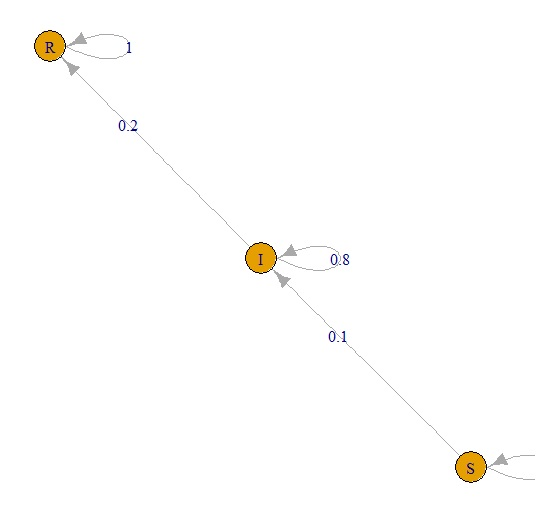But all we’ve done so far is to create our model. We haven’t yet done a simulation, which would show us how many people are in each of the three states as you move from one discrete timestep to many others. We can set up a data frame to contain labels for each timestep, and a count of how many people are in each state at each timestep. Then, we fill that data frame with the results after each timestep i, calculated by initialState*mcSIR^i:

```timesteps <- 100
sir.df <- data.frame( "timestep" = numeric(),
"S" = numeric(), "I" = numeric(),
"R" = numeric(), stringsAsFactors=FALSE)
for (i in 0:timesteps) {
newrow <- as.list(c(i,round(as.numeric(initialState * mcSIR ^ i),0)))
sir.df[nrow(sir.df) + 1, ] <- newrow
}```

Now that we have a data frame containing our SIR results (sir.df), we can display them to see what the values look like:

```> head(sir.df)
timestep  S  I  R
1        0 99  1  0
2        1 89 11  0
3        2 80 17  2
4        3 72 22  6
5        4 65 25 10
6        5 58 26 15
```

And then plot them to view our simulation results using this DTMC SIR Model:

```plot(sir.df\$timestep,sir.df\$S)
points(sir.df\$timestep,sir.df\$I, col="red")
points(sir.df\$timestep,sir.df\$R, col="green")```It’s also possible to use the markovchain package to identify elements of your system as it evolves over time:

```> absorbingStates(mcSIR)
 "R"
> transientStates(mcSIR)
 "S" "I"
S I R
[1,] 0 0 1
```

And you can calculate the first timestep that your Markov Chain reaches its steady state (the “time to absorption”), which your plot should corroborate:

```> ab.state <- absorbingStates(mcSIR)
> occurs.at <- min(which(sir.df[,ab.state]==max(sir.df[,ab.state])))
> (sir.df[row,]\$timestep)+1
 58
```

You can use this code to change the various transition probabilities to see what the effects are on the outputs yourself (sensitivity analysis). Also, there are methods you can use to perform uncertainty analysis, e.g. putting confidence intervals around your transition probabilities. We won’t do either of these here, nor will we create a Shiny app to run this simulation, despite the significant temptation.

## Simulation for Data Science With R

Hey everyone! I just wanted to give you the heads up on a book project that I’ve been working on (which should be available by Spring 2016). It’s all about using the R programming language to do simulation — which I think is one of the most powerful (and overlooked) tools in data science. Please feel free to email or write comments below if you have any suggestions for material you’d like to have included in it!

Originally, this project was supposed to be a secret… I’ve been working on it for about two years now, along with two other writing projects, and was approached in June by a traditional publishing company (who I won’t mention by name) who wanted to brainstorm with me about possibly publishing and distributing my next book. After we discussed the intersection of their wants and my needs, I prepared a full outline for them, and they came up with a work schedule and sent me a contract. While I was reading the contract, I got cold feet. It was the part about giving up “all moral rights” to my work, which sounds really frightening (and is not something I have to do under creative commons licensing, which I prefer). I shared the contract with a few colleagues and a lawyer, hoping that they’d say don’t worry… it sounds a lot worse than it really is. But the response I got was it sounds pretty much like it is.

While deliberating the past two weeks, I’ve been moving around a lot and haven’t been in touch with the publisher. I got an email this morning asking for my immediate decision on the matter (paraphrased, because there’s a legal disclaimer at the bottom of their emails that says “this information may be privileged” and I don’t want to violate any laws):

If we don’t hear from you, unfortunately we’ll be moving forward with this project. Do you still want to be on board?

The answer is YEAH – of COURSE I’m “on board” with my own project. But this really made me question the value of a traditional publisher over an indie publisher, or even self-publishing. And if they’re moving forward anyway, does that mean they take my outline (and supporting information about what I’m planning for each chapter) and just have someone else write to it? That doesn’t sound very nice. Since all the content on my blog is copyrighted by ME, I’m sharing the entire contents of what I sent to them on July 6th to establish the copyright on my outline in a public forum.

So if you see this chapter structure in someone ELSE’S book… you know what happened. The publisher came up with the idea for the main title (“Simulation for Data Science With R”) so I might publish under a different title that still has the words Simulation and R in them.

I may still publish with them, but I’ll make that decision after I have the full manuscript in place in a couple months. And after I have the chance to reflect more on what’s best for everyone. What do you think is the best route forward?

# Audience

Simulation is an essential (yet often overlooked) tool in data science – an interdisciplinary approach to problem-solving that leverages computer science, statistics, and domain expertise. This easy-to-understand introductory text for new and intermediate-level programmers, data scientists, and business analysts surveys five different simulation techniques (Monte Carlo, Discrete Event Simulation, System Dynamics, Agent-Based Modeling, and Resampling). The book focuses on practical and illustrative examples using the R Statistical Software, presented within the context of structured methodologies for problem solving (such as DMAIC and DMADV) that will enable you to more easily use simulation to make effective data-driven decisions. Readers should have exposure to basic concepts in programming but can be new to the R Statistical Software.

# Mission

This book helps its readers 1) formulate research questions that simulation can help solve, 2) choose an appropriate problem-solving methodology, 3) choose one or more simulation techniques to help solve that problem,  4) perform basic simulations using the R Statistical Software, and 5) present results and conclusions clearly and effectively.

# Objectives and achievements

• Learn about essential and foundational concepts in modeling and simulation
• Determine whether a simulation project is also a data science project
• Choose an appropriate problem-solving methodology for effective data-driven decision making
• Select suitable simulation techniques to provide insights about a given problem
• Build and interpret the results from basic simulations using the R Statistical Software

SECTION I: BASIC CONCEPTS

1. Introduction to Simulation for Data Science
2. Foundations for Decision-Making
3. SECRET NEW CHAPTER THAT YOU WILL BE REALLY EXCITED ABOUT

SECTION II: STOCHASTIC PROCESSES

1. Variation and Random Variable Generation
2. Distribution Fitting
3. Data Generating Processes

SECTION III: SIMULATION TECHNIQUES

1. Monte Carlo Simulation
2. Discrete Event Simulation
3. System Dynamics
4. Agent-Based Modeling
5. Resampling Methods
6. SECRET NEW CHAPTER THAT YOU WILL BE REALLY EXCITED ABOUT

SECTION IV: CASE STUDIES

1. Case Study 1: Possibly modeling opinion dynamics… specific example still TBD
2. Case Study 2: A Really Practical Application of Simulation (especially for women)

# Chapter 1: Introduction to Simulation for Data Science – 35 pages

## Description

This chapter explains the role of simulation in data science, and provides the context for understanding the differences between simulation techniques and their philosophical underpinnings.

BASIC

## Topics covered

Variation and Data-Driven Decision Making

What are Complex Systems?

What are Complex Dynamical Systems? What is systems thinking? Why is a systems perspective critical for data-driven decision making? Where do we encounter complex  systems in business or day-to-day life?

What is Data Science?

A Taxonomy of Data Science. The Data Science Venn Diagram. What are the roles of modeling and simulation in data science projects? “Is it a Data Science Project?” — a Litmus Test. How modeling and simulation align with data science.

What is a Model?

Conceptual Models. Equations. Deterministic Models, Stochastic Models. Endogeneous and Exogenous Variables.

What is Simulation?

Types of Simulation: Static vs. Dynamic, Stochastic vs. Deterministic, Discrete vs. Continuous, Terminating and Non-Terminating (Steady State). Philosophical Principles: Holistic vs. Reductionist, Kadanoff’s Universality, Parsimony, Sensitivity to Initial Conditions

Why Use Simulation?

Simulation and Big Data

Choosing the Right Simulation Technique

## Skills learned

The reader will be able to:

• Distinguish a model from a simulation
• Explain how simulation can provide a valuable perspective in data-driven decision making
• Understand how simulation fits into the taxonomy of data science
• Determine whether a simulation project is also a data science project
• Determine which simulation technique to apply to various kinds of real-world problems

# Chapter 2: Foundations for Decision Making – 25 pages

## Description

In this chapter, the reader will learn how to plan and structure a simulation project to aid in the decision-making process as well as the presentation of results. The social context of data science will be explained, emphasizing the growing importance of collaborative data and information sharing.

BASIC

## Topics covered

The Social Context of Data Science

Ethics and Provenance. Data Curation. Replicability, Reproducibility, and Open Science. Open, interoperable frameworks for collaborative data and information sharing. Problem-Centric Habits of Mind.

Selecting Key Performance Indicators (KPIs)

Determining the Number of Replications

Methodologies for Simulation Projects

A General Problem-Solving Approach

DMAIC

Root Cause Analysis (RCA)

PDSA

Verification and Validation Techniques

Output Analysis

## Skills learned

The reader will be able to:

• Plan a simulation study that is supported by effective and meaningful metadata
• Select an appropriate methodology to guide the simulation project
• Choose activities to ensure that verification and validation requirements are met
• Construct confidence intervals for reporting simulation output

# Chapter 3: Variability and Random Variate Generation – 25 pages

## Description

Simulation is powerful because it provides a way to closely examine the random behavior in systems that arises due to interdependencies and variability. This requires being able to generate random numbers and random variates that come from populations with known statistical characteristics. This chapter describes how random numbers and random variates are generated, and shows how they are applied to perform simple simulations.

MEDIUM

## Topics covered

Variability in Stochastic Processes

Why Generate Random Variables?

Pseudorandom Number Generation

Linear Congruential Generators

Inverse Transformation Method

Using sample for Discrete Distributions

Is this Sequence Random? Tests for Randomness

Autocorrelation, Frequency, Runs Tests. Using the randtests package

Tests for homogeneity

Simple Simulations with Random Numbers

## Skills learned

The reader will be able to:

• Generate pseudorandom numbers that are uniformly distributed
• Use random numbers to generate random variates from a target distribution
• Perform simple simulations using streams of random numbers

# Chapter 4: Data Generating Processes – 30 pages

## Description

To execute a simulation, you must be able to generate random variates that represent the physical process you are trying to emulate. In this chapter, we cover several common statistical distributions that can be used to represent real physical processes, and explain which physical processes are often modeled using those distributions.

MEDIUM

## Topics covered

What is a Data Generating Process?

Continuous, Discrete, and Multivariate Distributions

Discrete Distributions

Binomial Distribution

Geometric Distribution

Hypergeometric Distribution

Poisson Distribution

Continuous Distributions

Exponential Distribution

F Distribution

Lognormal Distribution

Normal Distribution

Student’s t Distribution

Uniform Distribution

Weibull Distribution

Chi2 Distribution

Stochastic Processes

Markov. Poisson. Gaussian, Bernoulli. Brownian Motion. Random Walk.

Stationary and Autoregressive Processes.

## Skills learned

The reader will be able to:

• Understand the characteristics of several common discrete and continuous data generating processes
• Use those distributions to generate streams of random variates
• Describe several common types of stochastic processes

# Chapter 5: Distribution Fitting – 30 pages

## Description

An effective simulation is driven by data generating processes that accurately reflect real physical populations. This chapter shows how to use a sample of data to determine which statistical distribution best represents the real population. The resulting distribution is used to generate random variates for the simulation.

MEDIUM

## Topics covered

Why is Distribution Fitting Essential?

Techniques for Distribution Fitting

Shapiro-Wilk Test for Normality

Anderson-Darling Test

Lillefors Test

Kolmogorov-Smirnov Test

Chi2 Goodness of Fit Test

Other Goodness Of Fit Tests

When There’s No Data, Use Interviews

## Skills learned

The reader will be able to:

• Use a sample of real data to determine which data generating process is required in a simulation
• Transform data to find a more effective data generating process
• Estimate appropriate distributions when samples of real data are not available

# Chapter 6: Monte Carlo Simulation – 30 pages

## Description

This chapter explains how to set up and execute simple Monte Carlo simulations, using data generating processes to represent random inputs.

## Topics covered

Anatomy of a Monte Carlo Project

The Many Flavors of Monte Carlo

The Hit-or-Miss Method

Example: Estimating Pi

Monte Carlo Integration

Example: Numerical Integration of y = x2

Estimating Variables

Monte Carlo Confidence Intervals

Example: Projecting Profits

Sensitivity Analysis

Example: Projecting Variability of Profits

Example: Projecting Yield of a Process

Markov Chain Monte Carlo

## Skills learned

The reader will be able to:

• Plan and execute a Monte Carlo simulation in R
• Construct confidence intervals using the Monte Carlo method
• Determine the sensitivity of process outputs and interpret the results

# Chapter 7: Discrete Event Simulation – 30 pages

## Topics covered

Anatomy of a DES Project

Entities, Locations, Resources and Events

System Performance Metrics

Queuing Models and Kendall’s Notation

The Event Calendar

Manual Event Calendar Generation

Example: An M/M/1 system in R

Using the queueing package

Using the simmer package

Arrival-Counting Processes with the NHPoisson Package

Survival Analysis with the survival Package

Example: When Will the Bagels Run Out?

## Skills learned

The reader will be able to:

• Plan and execute discrete event simulation in R
• Choose an appropriate model for a queueing problem
• Manually generate an event calendar to verify simulation results
• Use arrival counting processes for practical problem-solving
• Execute a survival analysis in R and interpret the results

# Chapter 8: System Dynamics – 30 pages

## Description

This chapter presents system dynamics, a powerful technique for characterizing the effects of multiple nested feedback loops in a dynamical system. This technique helps uncover the large-scale patterns in a complex system where interdependencies and variation are critical.

## Topics covered

Anatomy of a SD Project

The Law of Unintended Consequences and Policy Resistance

Introduction to Differential Equations

Causal Loop Diagrams (CLDs)

Stock and Flow Diagrams (SFDs)

Using the deSolve Package

Example: Lotka-Volterra Equations

Dynamic Archetypes

Linear Growth

Exponential Growth and Collapse

S-Shaped Growth

S-Shaped Growth with Overshoot

Overshoot and Collapse

Delays and Oscillations

Using the stellaR and simecol Packages

## Skills learned

The reader will be able to:

• Plan and execute a system dynamics project
• Create causal loop diagrams and stock-and-flow diagrams
• Set up simple systems of differential equations and solve them with deSolve in R
• Predict the evolution of stocks using dynamic archetypes in CLDs
• Convert STELLA models to R

# Chapter 9: Agent-Based Modeling – 25 pages

## Description

Agent-Based Modeling (ABM) provides a unique perspective on simulation, illuminating the emergent behavior of the whole system by simply characterizing the rules by which each participant in the system operates. This chapter provides an overview of ABM, compares and contrasts it with the other simulation techniques, and demonstrates how to set up a simulation using an ABM in R.

## Topics covered

Anatomy of an ABM Project

Emergent Behavior

PAGE (Percepts, Actions, Goals, and Environment)

Turtles and Patches

Using the RNetLogo package

## Skills learned

The reader will be able to:

• Plan and execute an ABM project in R
• Create specifications for the ABM using PAGE

# Chapter 10: Resampling – 25 pages

## Description

Resampling methods are related to Monte Carlo simulation, but serve a different purpose: to help us characterize a data generating process or make inferences about the population our data came from when all we have is a small sample. In this chapter, resampling methods (and some practical problems that use them) are explained.

MEDIUM

## Topics covered

Anatomy of an Resampling Project

Bootstrapping

Jackknifing

Permutation Tests

## Skills learned

The reader will be able to:

• Plan and execute a resampling project in R
• Understand how to select and use a resampling technique for real data

# Chapter 11: Comparing the Simulation Techniques – 15 pages

## Description

In this chapter, the simulation techniques will be compared and contrasted in terms of their strengths, weaknesses, biases, and computational complexity.

## Topics covered

TBD – at least two simulation approaches will be applied

## Skills learned

The reader will learn how to:

• Think about a simulation study holistically
• Select an appropriate combination of techniques for a real simulation study

## Logistic Growth, S Curves, Bifurcations, and Lyapunov Exponents in R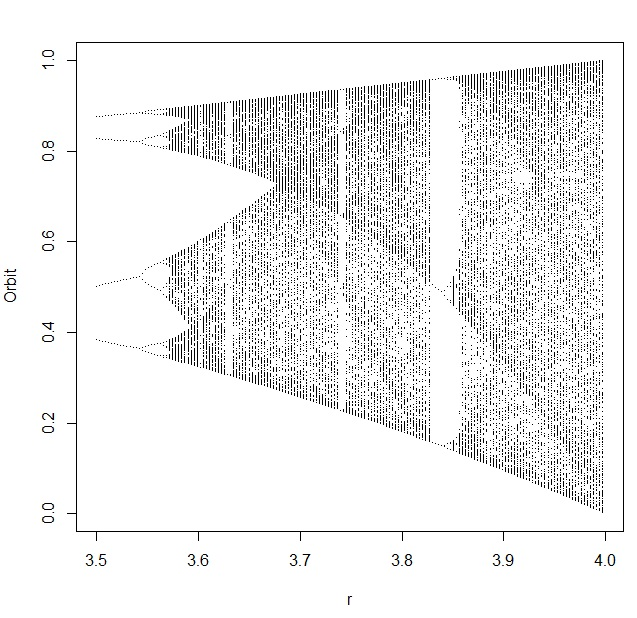If you’ve ever wondered how logistic population growth (the Verhulst model), S curves, the logistic map, bifurcation diagrams, sensitive dependence on initial conditions, “orbits”, deterministic chaos, and Lyapunov exponents are related to one another… this post explains it in just 10 steps, each with some code in R so you can explore it all yourself. I’ve included some code written by other people who have explored this problem (cited below) as portions of my own code.

It all starts with a hypothesized population… and a process where the size of the population changes over time. We want to understand how (and under what conditions) those changes occur, so we choose a model that characterizes population changes: the logistic growth model. It’s been used in biology, ecology, econometrics, marketing, and other areas.

1. The logistic growth model describes how the size of a population (N) changes over time (t), based on some maximum population growth rate (r). There is a limiting factor called the carrying capacity (K) which represents the total population that the environment could support, based on the amount of available resources. dN/dt is the rate of change of the population over time.2. You can simplify the logistic growth model by defining a new variable x to represent the portion of the population that’s alive, compared to the total population that the environment could support (and keep alive). So with x = N/K, you get a new differential equation in terms of x. Now we are looking at the rate of change of the population fraction over time. Once x = N/K = 1, the environment can’t support any more members in the population: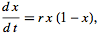3. You can solve this equation by integration! Then, you’ll have an expression that you can use to calculate x (which is still the population fraction) for any time t. This is called the sigmoid or (more commonly), the S Curve. To compute x at any time t, all we need to know is how big the population was when we started looking at it (x0) and the maximum growth rate r:4. The equation for the S Curve is deterministic and continuous. If we want to solve it numerically, we have to discretize it by chopping up that continuous axis that contains time into little tiny pieces of time. That’s what produces the difference equation that we recognize as the logistic map. It’s a map because it “maps” each value of the sequence onto the next value in the sequence. As long as you know one of those values for x (indicated by the subscript n), you’ll be able to figure out the next value of x (indicated by the subscript n+1). The value x[n] is the population fraction of the current generation, and the value x[n+1] is the population fraction for the next generation. This makes the logistic map a Markov chain. If you plot x[n] on the x axis and x[n+1] on the y axis, this expression will produce the familiar upside down parabola: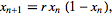5. The logistic map behaves differently depending upon the maximum growth rate (r) that describes your population. This parameter is also called fecundity and represents how rabbit-like your population is reproducing. The higher the r, the more productive, like rabbits (although I’m not sure precisely which r you’d choose if you were studying rabbits). Here is an R function that you can use to generate the last M iterations from a sequence of N total, developed and described at Mage’s Blog:

```logistic.map <- function(r, x, N, M) {
## from http://www.magesblog.com/2012/03/logistic-map-feigenbaum-diagram.html
## r: bifurcation parameter
## x: initial value, something greater than 0 and less than 1
## N: number of iterations (total)
## M: number of iteration points to be returned
z <- 1:N
z <- x
for(i in c(1:(N-1))){
z[i+1] <- r *z[i] * (1 - z[i])
}
## Return the last M iterations
z[c((N-M):N)]
}```

6. The logistic map has many interesting properties, but here are two in particular (the first in Step 6 and the second in Step 7). First, for several values you can choose for r, the chain converges to a single value (or fixed point) when n gets really big. For other values of r, the value of x will eventually bounce between two values instead of converging (a limit cycle of 2). For other values of r, the value of x will eventually bounce between four values instead of converging. Sometimes, x will bounce around a near limitless collection of values (a condition called deterministic chaos). The eventual values (or collection of eventual values, if they bounce between values) is called an orbit. For example, when the growth rate r is 2.6, the logistic map rapidly converges to an orbit of about 0.615:

`plot(logistic.map(2.6,.01,20,20), type="l")`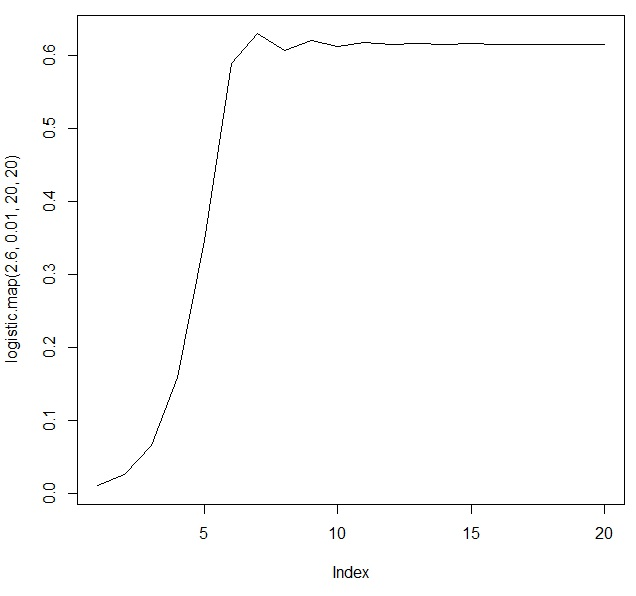7. Sometimes, it can be nice to take a look at how the values bounce around, and where they eventually converge (or not). To do this, we use cobweb diagrams (which are also sometimes called web diagrams). I used a function that I found at http://bayesianbiologist.com to plot the behavior of the orbits for r=2.6, r=3.2, and r=3.9:

```logistic.cobweb <- function(r) {
x<-seq(0,1,length=100)
x_next <- lapply(2:N, function(i) r*x[i]*(1-x[i]))
plot(x[2:N],x_next,type="l",xlim=c(0,1), ylim=c(0,1), main=paste("r=",r),
xlab=expression(x[t]),ylab=expression(x[t+1]), col="red", lwd=2)
abline(0,1)

start=runif(1,0,1)
vert=FALSE
lines(x=c(start,start),y=c(0,r*start*(1-start)) )
for(i in 1:(2*N)) {
if(vert) {
lines(x=c(start,start),y=c(start,r*start*(1-start)) )
vert=FALSE
} else {
lines(x=c(start, r*start*(1-start)), y=c(r*start*(1-start), r*start*(1-start)) )
vert=TRUE
start=r*start*(1-start)
}
}
}

par(mfrow=c(1,3))
logistic.cobweb(2.6)
logistic.cobweb(3.3)
logistic.cobweb(3.9)```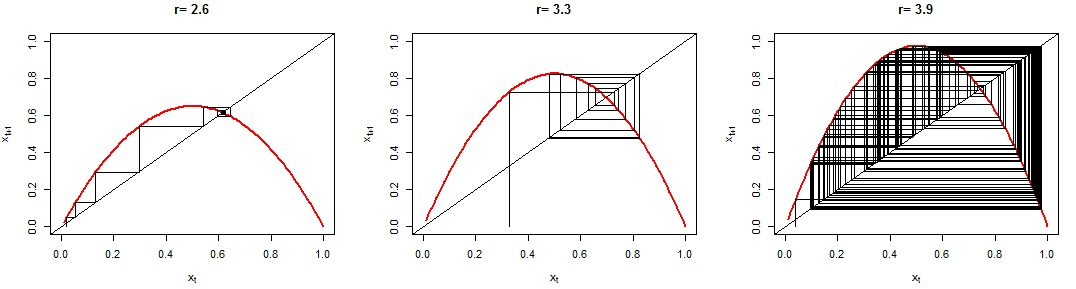8. (Remember to dev.off() before you continue.) Second, for some values of r, the logistic map shows sensitive dependence on initial conditions. For example, let’s see what happens for two different growth rates (r=3 and r=3.9) when we start one iteration with an x[n]  of 0.5 COLORED BLACK, and another one with an x[n] of 0.5001 COLORED RED. It’s a small, small difference that can lead to big, BIG variations in the orbits. In the r=3 case, the chain produced by the logistic map with x[n] of 0.5 (in black) is IDENTICAL to the chain produced by the logistic map with x[n] of 0.5001 (in red). That’s why you can’t see the black… the values are the same! But for the r=3.9 case, the chain produced by the logistic map with x[n] of 0.5 (in black) RAPIDLY DIVERGES from the chain produced by the logistic map with x[n] of 0.5001 (in red). They are very different, despite a very tiny difference in initial conditions! The logistic map for r=3.9 shows a very sensitive dependence on initial conditions

```par(mfrow=c(2,1))
first <- logistic.map(3,.5,120,100)
second <- logistic.map(3,.5001,120,100)
plot(1:length(first),first,type="l",main="r=3 is not sensitive to initial conditions")
lines(1:length(second),second,type="l",col="red")
first <- logistic.map(3.9,.5,120,100)
second <- logistic.map(3.9,.5001,120,100)
plot(1:length(first),first,type="l",main="but r=3.9 is EXTREMELY sensitive")
lines(1:length(second),second,type="l",col="red")

```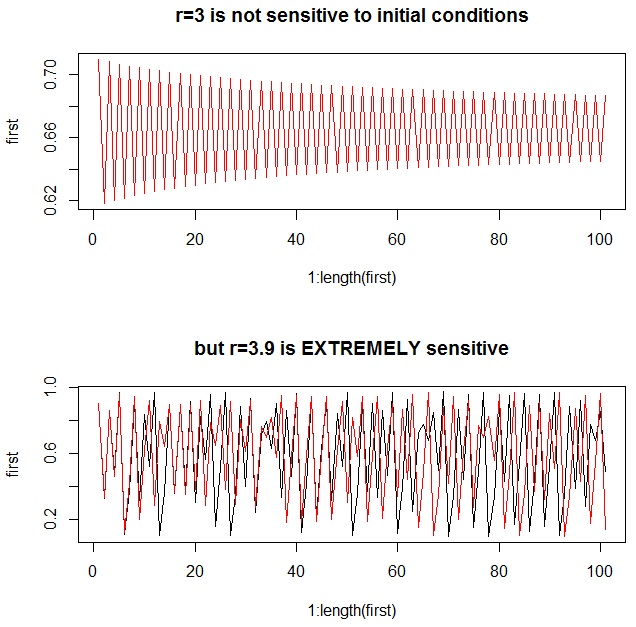9. For any chain, we can determine just how sensitive the logistic map is to initial conditions by looking at the Lyapunov exponent. Very simplistically, if the Lyapunov exponent is negative, the chain will converge to one or more fixed points for that value of r. If the Lyapunov exponent is positive, the chain will demonstrate deterministic chaos for that value of r. If the Lyapunov exponent is zero, there is a bifurcation: a 1-cycle is doubling to a 2-cycle, a 2-cycle is doubling to a 4-cycle, or so forth. The top chart shows an approximation of the Lyapunov exponent based on the first 500 iterations (ideally, you’d use an infinite number, but that would eat up too much computing time), and the bottom chart shows a bifurcation diagramYou’ll notice that the Lyapunov exponents are zero where a bifurcation occurs. To interpret the bifurcation diagram, just remember that each vertical slice through it represents the results of ONE COMPLETELY CONVERGED CHAIN from the logistic map. So it shows the results from many, many, many completely converged chains – and provides an excellent way for us to look at the behavior of MANY different types of populations in just one chart:

```n <- 400
XI <- lya <- 0
x <- seq(0,4,0.01)
for (i in 1:n) {
xi <- logistic.map(x[i],.01,500,500)
XI <- rbind(XI,xi)
}
for (i in 1:length(x)) {
lya[i] <- sum(log(abs(x[i]-(2*x[i]*XI[i,]))))/length(x)
}
plot(x,lya,ylim=c(-4,1),xlim=c(0,4),type="l",main="Lyapunov Exponents for Logistic Map")
abline(h=0, lwd=2, col="red")
# next 3 lines from http://www.magesblog.com/2012/03/logistic-map-feigenbaum-diagram.html:
my.r <- seq(0, 4, by=0.003)
Orbit <- sapply(my.r, logistic.map, x=0.1, N=1000, M=300)
r <- sort(rep(my.r, 301))

par(mfrow=c(2,1))
plot(x,lya,ylim=c(-5,1),xlim=c(0,4),type="l",main="Lyapunov Exponents for Logistic Map")
abline(h=0, col="red", lwd=2)
abline(v=3, col="blue", lwd=2)
plot(r, Orbit, pch=".", cex=0.5, main="Bifurcation Diagram for r=0 to r=4 Logistic Maps")
abline(v=3, col="blue", lwd=2)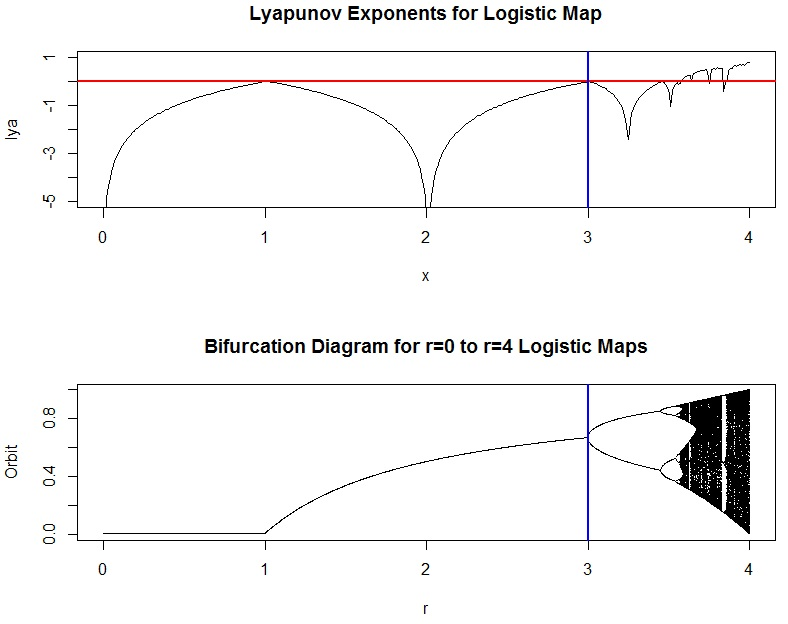```

10. Notice that in the bifurcation diagram, we can easily see that when r is between 0 and 1, the population converges to extinction. This makes sense, because the growth rate is smaller than what’s required to sustain the size of the population. You might like to zoom in, though, and see what the orbits look like for some smaller portions of the diagram. Here’s how you can do it (but be sure to refresh your graphics window with dev.off() before you try it). Try changing the plot character (pch) too, or maybe the size of the characters with cex=0.2 or cex=0.5 in the last line:

```# adapted from http://www.magesblog.com/2012/03/logistic-map-feigenbaum-diagram.html:
my.r <- seq(3.5, 4, by=0.003)
Orbit <- sapply(my.r, logistic.map, x=0.1, N=1000, M=300)
multiplier <- length(Orbit)/length(my.r)
r <- sort(rep(my.r, multiplier))
plot(r, Orbit, pch=".")```That’s it!

Find out more information on these other web pages, which are listed in order of difficulty level:

## A Linear Congruential Generator (LCG) in R

In my simulation classes, we talk about how to generate random numbers. One of the techniques we talk about is the Linear Congruential Generator (LCG). Starting with a seed, the LCG produces the first number in the sequence, and then uses that value to generate the second one. The second value is used to generate the third, the third to generate the fourth, and so on. The equation looks like this: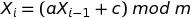where a is a multiplier, c is a shift, and m is a modulus. (Modulus just means “find me the remainder when you divide the stuff to the left of the mod operator by the stuff to the right”. Fortunately there is an easy way to do this in R: a mod b is expressed as a %% b.) When you use the LCG to generate a stream of random numbers, they will always be between 0 and (m-1), and the sequence will repeat every m occurrences. When you take the sequence of values x and divide it by the mod m, you get uniformly distributed random numbers between 0 and 1 (but never quite hitting 1). The LCG is a pseudorandom number generator because after a while, the sequence in the stream of numbers will begin to repeat.

I wrote an exam last week with some LCG computations on it, but then I got lazy and didn’t want to do the manual calculations… so I wrote a function in R. Here it is. It returns two different vectors: the collection of numbers between 0 and (m – 1) that the LCG produces, and the collection of uniformly distributed random numbers between 0 and 1 that we get by dividing the first collection by m:

```lcg <- function(a,c,m,run.length,seed) {
x <- rep(0,run.length)
x <- seed
for (i in 1:(run.length-1)) {
x[i+1] <- (a * x[i] + c) %% m
}
U <- x/m # scale all of the x's to
# produce uniformly distributed
# random numbers between [0,1)
return(list(x=x,U=U))
}```

So for example, if your LCG looks like this: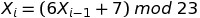Then, after loading in the function above, you could choose a seed (say, 5) and generate a stream of 20 random numbers:

```> z <- lcg(6,7,23,20,5)
> z
\$x
 5 14 22 1 13 16 11 4 8 9 15 5 14 22 1 13 16 11
 4 8

\$U
 0.21739130 0.60869565 0.95652174 0.04347826 0.56521739
 0.69565217 0.47826087 0.17391304 0.34782609 0.39130435
 0.65217391 0.21739130 0.60869565 0.95652174 0.04347826
 0.56521739 0.69565217 0.47826087 0.17391304 0.34782609```

The values contained within z\$x are the numbers that the LCG produces, and the values contained within z\$U are the values of z\$x scaled to fall between 0 and 1. Both z\$x and z\$U are uniformly distributed, although the pattern will become more apparent as you generate more and more random numbers in your stream. Here’s what they look like with 10000 numbers in the stream:

```> z <- lcg(6,7,23,10000,5)
> par(mfrow=c(1,2))
> hist(z\$x,col="red")
> hist(z\$U,col="purple")```But how good is your random number generator? First, you know you want a large (and preferably odd valued mod). That way, the numbers will not repeat so quickly. But there are also considerations dealing with what multiplier and shift you should use. I like checking out the quality of my random number generator by plotting how they move in sequence around a two dimensional grid: the better the random number generator, the more filling of the space you will see.

You can play around with these plots to find a “good” random number generator:

```> z1 <- lcg(6,7,23,2000,5) # "Bad" LCG
> z2 <- lcg(1093,18257,86436,2000,12) # "Good" LCG
> par(mfrow=c(1,2))
> plot( z1\$U[1:(length(z1\$U)-1)], z1\$U[2:length(z1\$U)] , main="Bad")
> plot( z2\$U[1:(length(z2\$U)-1)], z2\$U[2:length(z2\$U)] , main="Good")```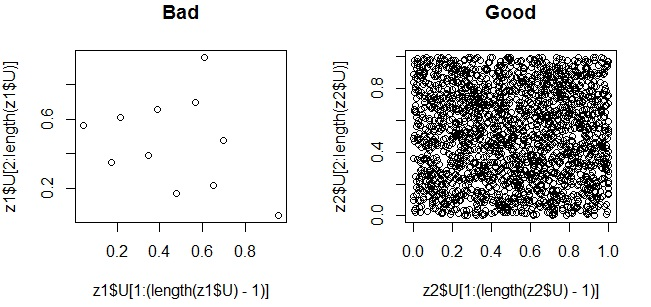Although there are many heuristics you can use to avoid creating a “bad” random number generator, it’s really difficult to design a “good” one. People make careers out of this. I don’t claim to be an expert, I just know how to test to see if a random number generator is suitable for my simulations. (I also really like the handout at http://www.sci.csueastbay.edu/~btrumbo/Stat3401/Hand3401/CongGenIntroB.pdf that explains this graphing approach more, and also talks about how you have to look at patterns in three dimensions as well. When I was searching around for a “good LCG that would give me a nice graph” I found this handout.)

## Warren Buffett on Simulation & Modeling

John Hunter shared some excerpts from Warren Buffett’s 2009 Letter to Shareholders. I particularly liked this one part where he reflects on the outcomes of economic modeling and forecasting:

Investors should be skeptical of history-based models. Constructed by a nerdy-sounding priesthood using esoteric terms such as beta, gamma, sigma and the like, these models tend to look impressive. Too often, though, investors forget to examine the assumptions behind the symbols. Our advice: Beware of geeks bearing formulas.

I’d like to amend this: Beware of geeks bearing formulas who a) can’t tell you what every part of the derivation means, b) don’t know the model’s underlying assumptions, and c) don’t know what “threats to validity” are. (And if you’re the geek in question, be able to explain how your models and forecasts work!!)

Models can be a great way to capture the dynamics of social and technical systems, and simulations can help us explore how these systems will evolve over time – but how those models are initialized, and the simplifying assumptions they use to generate results, are just as important as the answers they propose.